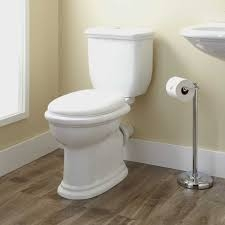# Half-filled 7336

The water tank is filled with the first inflow in one hour and 10 minutes and the second in 60 minutes. How many minutes will the tank be half-filled with both inlets if the second outlet is opened 12 minutes later?

t =  6 min

### Step-by-step explanation:Did you find an error or inaccuracy? Feel free to write us. Thank you!

Tips for related online calculators
Looking for calculator of harmonic mean?
Looking for a statistical calculator?
Do you have a linear equation or system of equations and looking for its solution? Or do you have a quadratic equation?